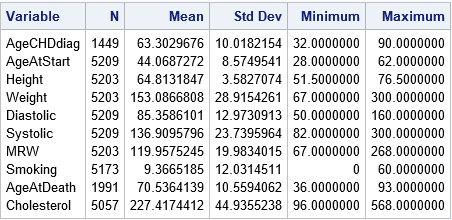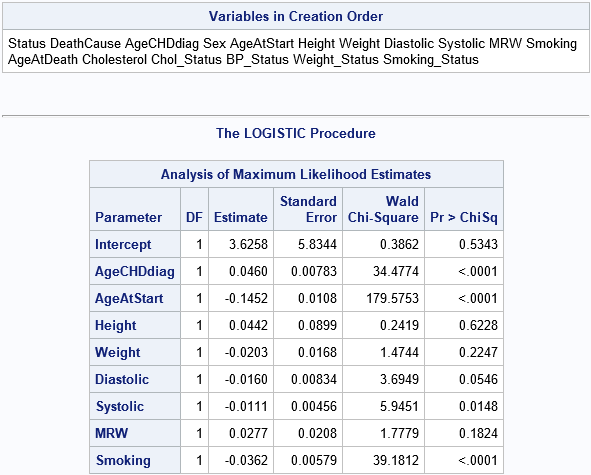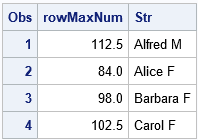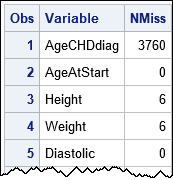The SAS language provides syntax that enables you to quickly specify a list of variables. SAS statements that accept variable lists include the KEEP and DROP statements, the ARRAY statement, and the OF operator for comma-separated arguments to some functions. You can also use variable lists on the VAR statements and MODEL statements of analytic procedures.

This article describes six ways to specify a list of variables in SAS. There is a section in the SAS documentation that describes how to construct lists, but this blog post provides more context and a cut-and-paste example for every syntax. This article demonstrates the following:

• Use the _NUMERIC_, _CHARACTER_, and _ALL_ keywords to specify variables of a certain type (numeric or character) or all types.
• Use a single hyphen (-) to specify a range of variables that have a common prefix and a sequential set of numerical suffixes.
• Use the colon operator (:) to specify a list of variables that begin with a common prefix.
• Use a double-hyphen (--) to specify a consecutive set of variables, regardless of type. You can also use a variation of this syntax to specify a consecutive set of variables of a certain type (numeric or character).
• Use the OF operator to specify variables in an array or in a function call.
• Use macro variables to specify variables that satisfy certain characteristics.

Some companies might discourage the use of variable lists in production code because automated lists can be volatile. If the number and names of variables in your data sets occasionally change, it is safer to manually list the variables that you are analyzing. However, for developing code and constructing examples, lists can be a huge time saver.

### Use the _NUMERIC_, _CHARACTER_, and _ALL_ keywords

You can specify all numeric variables in a data set by using the _NUMERIC_ keyword. You can specify all character variables by using the _CHARACTER_ keyword. Many SAS procedures use a VAR statement to specify the variables to be analyzed. When you want to analyze all variables of a certain type, you can use these keywords, as follows:

```/* compute descriptive statistics of allnumeric variables */ proc means data=Sashelp.Heart nolabels; var _NUMERIC_; /* _NUMERIC_ is the default */ run;   /* display the frequencies of all levels for all character variables */ proc freq data=Sashelp.Heart; tables _CHARACTER_; /* _ALL_ is the defaul */ run;```One of my favorite SAS programming tricks is to use these keywords in a KEEP or DROP statement (or data set option). For example, the following statements create a new data set that contains all numeric variables and two character variables from the Sashelp.Heart data:

```data HeartNumeric; set Sashelp.Heart(keep=_NUMERIC_ /* all numeric variables */ Sex Smoking_Status); /* two character variables */ run;```

An example of using the _ALL_ keyword is shown in the section that discusses the OF operator.

### Use a hyphen to specify numerical suffixes

In many situations, variables are named with a common prefix and numerical suffix. For example, financial data might have variables that are named Sales2008, Sales2009, ..., Sales2017. In simulation studies, variables often have names such as X1, X2, ..., X50. The hyphen enables you to specify the first and last variable in a list. The first example can be specified as Sales2008-Sales2017. The second example is X1-X50.

The following DATA step creates 10 variables, including the variables x1-x6. Notice that the data set variables are not in alphanumeric order. That is okay. The syntax x1-x6 will select the six variables x1, x2, x3, x4, x5, and x6 regardless of their physical order in the data. The call to PROC REG uses the six variables in a linear regression:

```data A; retain Y x1 x3 Z x6 x5 x2 W x4 R; /* create 10 variables and one observation. Initialize to 0 */ run; proc reg data=A plots=none; model Y = x1-x6; run;```

The parameter estimates from PROC REG are displayed in the order that you specify in the MODEL statement. However, if you use the SET statement in a DATA step, the variables appear in the original order unless you intentionally reorder the variables:

```data B; set A(keep=x1-x6); run;```

### Use the colon operator to specify a prefix

If you want to use variables that have a common prefix but have a variety of suffixes, you can use the colon operator (:), which is a wildcard character that matches any name that begins with a specified prefix. For example, the following DATA step creates a data set that contains 10 variables, including five variables that begin with the prefix 'Sales'. The subsequent DATA step drops the variables that begin with the prefix 'Sales':

```data A; retain Sales17 Y Sales16 Z SalesRegion Sales_new Sales1 R; /* 1 obs. Initialize to 0 */ run;   data B; set A(drop= Sales: ); /* drop all variables that begin with 'Sales' */ run;```

### Use a double-hyphen to specify consecutive variables

The previous sections used wildcard characters to match variables that had a specified type or prefix. In the previous sections, you will get the same set of variables regardless of how they might be ordered in the data set. You can use a double-hyphen (--) to specify a consecutive set of variables. The variables you get depend on the order of the variables in the data set.

```data A; retain Y 0 x3 2 C1 'A' C2 'BC' Z 3 W 4 C4 'D' C5 'EF'; /* Initialize eight variables */ run; data B; set A(keep=x3--C4); run;```

In this example, the data set B contains the variables x3, C1, C2, Z, W, and C4. If you use the double-hyphen to specify a list, be sure that you know the order of the variables and that this order is never going to change. If the order of the variables changes, your program will behave differently.

You can also specify all variables of a certain type within a range of variables. The syntax Y-numeric-Z specifies all numeric variables between Y and Z in the data set. The syntax Y-character-Z specifies all character variables between Y and Z. For example, the following call to PROC CONTENTS displays the variables (in order) in the Sashelp.Heart data. The call to PROC LOGISTIC specifies all the numeric variables between (and including) the AgeCHDiag variable and the Smoking variable:

```proc contents data=Sashelp.Heart order=varnum ; run;   proc logistic data=Sashelp.Heart; model status = AgeCHDdiag-numeric-Smoking; ods select ParameterEstimates; run;```### Arrays and the OF operator

You can use variable lists to assign an array in a SAS DATA step. For example, the following program creates a numerical array named X and a character array named C. The program finds the maximum value in each row and puts that value into the variable named rowMaxNUm. The program also creates a variable named Str that contains the concatenation of the character values for each row:

```data Arrays; set sashelp.Class; array X {*} _NUMERIC_; /* X is 1st var, X is 2nd var, etc */ array C {*} _CHARACTER_; /* C is 1st var, C is 2nd var, etc */ /* use the OF operator to pass values in array to functions */ rowMaxNum = max(of x[*]); /* find the max value in this array (row) */ length Str \$30; call catx(' ', Str, of C[*]); /* concatenate the strings in this array (row) */ keep rowMaxNum Str; run;   proc print data=Arrays(obs=4); run;```You can use the OF operator directly in functions without creating an array. For example, the following program uses the _ALL_ keyword to output the "complete cases" for the Sashelp.Heart data. The program drops any observation that has a missing value for any variable:

```data CompleteCases; set Sashelp.Heart; if cmiss(of _ALL_)=0; /* output only complete cases for all vars */ run;```

### Use macro variables to specify a list

The previous sections demonstrate how you can use syntax to specify a list of variables to SAS statements. In contrast, this section describes a technique rather than syntax. It is sometimes the case that the names of variables are in a column in a data set. There might be other columns in the data set that contain characteristics or statistics for the variables. For example, the following call to PROC MEANS creates an output data set (called MissingValues) that contains columns named Variable and NMiss.

```proc means data=Sashelp.Heart nolabels NMISS stackodsoutput; var _NUMERIC_; ods output Summary = MissingValues; run; proc print; run;```Suppose you want to keep or drop those variables that have one or more missing values. The following PROC SQL call creates a macro variable (called MissingVarList) that contains a space-separated list of all variables that have at least one missing value. This technique has many applications and is very powerful.

```/* Use PROC SQL to create a macro variable (MissingVarList) that contains the list of variables that have a property such as missing values */ proc sql noprint; select Variable into :MissingVarList separated by ' ' from MissingValues where NMiss > 0; quit; %put &=MissingVarList;```
`MISSINGVARLIST=AgeCHDdiag Height Weight MRW Smoking AgeAtDeath Cholesterol`

You can now use the macro variable in a KEEP, DROP, VAR, or MODEL statement, such as KEEP=&MissingVarList;

### Summary

This article shows six ways to specify a list of variables to SAS statements and functions. The SAS syntax provides keywords (_NUMERIC_, _CHARACTER_, and _ALL_) and operators (hyphen, colon, and double-hyphen) to make it easy to specify a list of variables. You can use the syntax in conjunction with the OF operator to pass a variable list to some SAS functions. Lastly, if the names of variables are stored in a column in a data set, you can use the full power of PROC SQL to create a macro variable that contains variables that satisfy certain criteria.

Do you use shorthand syntax to specify lists of variables? Why or why not? Leave a comment.

The post 6 easy ways to specify a list of variables in SAS appeared first on The DO Loop.